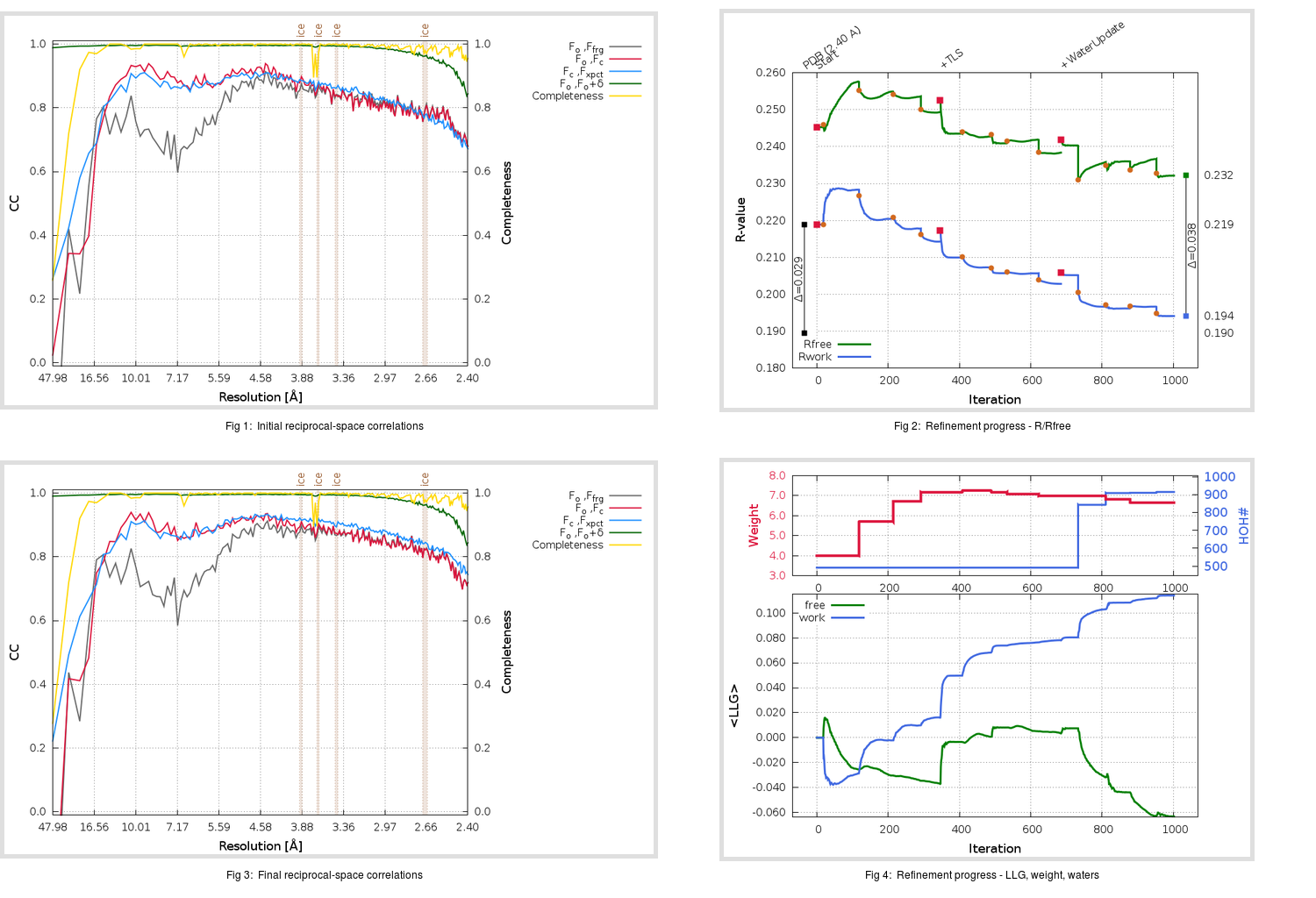Content:

```    Diffraction limits & principal axes of ellipsoid fitted to diffraction cut-off surface:
2.402         1.0000   0.0000   0.0000       a*
2.331         0.0000   1.0000   0.0000       b*
2.523         0.0000   0.0000   1.0000       c*
```

## Deposited

` `
 Date deposited Date data collection Resolution R, Rfree 20151118 2.40 0.1879 0.2188

Molprobity (CCP4 7.0 version) summary:

```Ramachandran outliers =   0.06 %
favored =  96.91 %
Rotamer outliers      =   4.77 %
C-beta deviations     =     0
Clashscore            =   5.26
RMS(bonds)            =   0.0046
RMS(angles)           =   0.88
MolProbity score      =   1.98
Resolution            =   2.40
R-work                =   0.1879
R-free                =   0.2188
```

```Number of waters      =   492

<B> (all atoms) =   67.38 ( sd =   24.05 ) for      13810 non-hydrogen atoms
<B>   (protein) =   67.48 ( sd =   24.18 ) for      13123 non-hydrogen atoms
<B>     (water) =   56.94 ( sd =   10.21 ) for        492 non-hydrogen atoms
<B>    (others) =   87.18 ( sd =   26.66 ) for        195 non-hydrogen atoms

B min/max       (all non-hydrogen atoms) =   16.85 /  228.03
B min/max   (protein non-hydrogen atoms) =   16.85 /  228.03
B min/max     (water non-hydrogen atoms) =   24.14 /   79.09
B min/max     (other non-hydrogen atoms) =   33.37 /  153.61
```

## BUSTER (re-)refinement

` `

Molprobity (CCP4 7.0 version) summary:

```Ramachandran outliers =   0.12 %
favored =  97.21 %
Rotamer outliers      =   4.63 %
C-beta deviations     =     3
Clashscore            =   3.98
RMS(bonds)            =   0.0117
RMS(angles)           =   1.54
MolProbity score      =   1.83
Resolution            =   2.40
R-work                =   0.1941
R-free                =   0.2322
```

```Number of waters      =   915

<B> (all atoms) =   57.32 ( sd =   19.51 ) for      14233 non-hydrogen atoms
<B>   (protein) =   57.20 ( sd =   19.77 ) for      13123 non-hydrogen atoms
<B>     (water) =   55.93 ( sd =   13.19 ) for        915 non-hydrogen atoms
<B>    (others) =   72.26 ( sd =   20.96 ) for        195 non-hydrogen atoms

B min/max       (all non-hydrogen atoms) =   15.30 /  134.09
B min/max   (protein non-hydrogen atoms) =   15.30 /  134.09
B min/max     (water non-hydrogen atoms) =   18.28 /  123.82
B min/max     (other non-hydrogen atoms) =   29.11 /  109.80
```

Refinement progression:Results:

` `
 File Remark 5FO8_aB_refine.01_03_refine.pdb.gz exact refinement commands are in header 5FO8_aB_refine.01_03_refine.mtz.gz including original deposited data and several re-refinement map coefficients 5FO8_aB_refine.01_03_BUSTER_model.cif.gz including any non-standard compound restraints 5FO8_aB_refine.01_03_BUSTER_refln.cif.gz